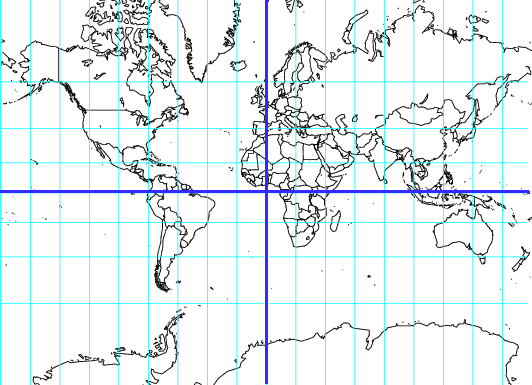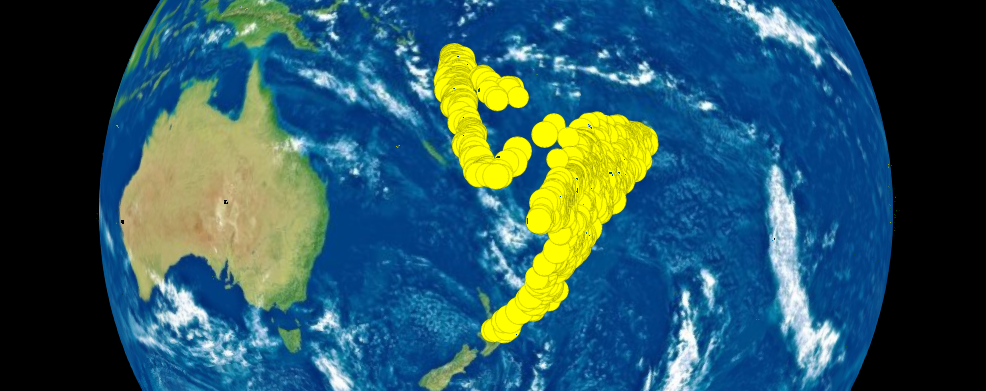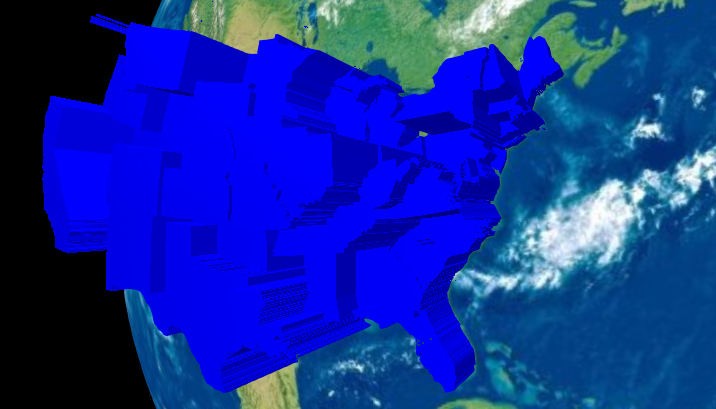#webglobe: Interactive 3D Maps

You want to understand your data, but it's spatially distributed and you're afraid that trying to make sense of it on something gross, like a Mercator projection, is going to lead you to bad intuitions.(Greenland is nowhere near that big in reality.)

webglobe can help you do this! It allows you to interactively visualize your data on either a three-dimensional globe or a flat map.

#Example: Earth quakes

``````library(webglobe)              #Load the library
data(quakes)                   #Load up some data

wg <- webglobe(immediate=TRUE) #Make a webglobe (should open a net browser)
Sys.sleep(10)                     #Wait for browser to start, or it won't work
wg + wgpoints(quakes\$lat, quakes\$lon, size=5*quakes\$mag) #Plot quakes
wg + wgcamcenter(-24, 178.0650, 8000)                    #Move camera
``````#Example: States

``````library(webglobe)                 #Load the library
m  <- ggplot2::map_data("state")  #Get data
m\$extrude_height <- 1000000*runif(nrow(m),min=0,max=1)
wg <- webglobe(immediate=FALSE)    #Make a webglobe (should open a net browser)
wg <- wg + wgpolygondf(m,fill="blue",alpha=1,stroke=NA)
wg
``````#Example: dggridR

dggridR is a package for binning spatial data into equally-sized hexagonal or triangular cells. It makes spatial analysis and statistics easier by solving the problem of having to worry about whether your projection is appropriate for the region you are using: all cells have the same area. It also works well with webglobe!

``````library(dggridR)
library(dplyr)
library(webglobe)
library(colorspace)

#Construct a global grid with cells approximately 1000 miles across
dggs          <- dgconstruct(type="ISEA4T",spacing=1000, metric=FALSE, resround='down')

#Load included test data set
data(dgquakes)

#Get the corresponding grid cells for each earthquake epicenter (lat-long pair)
dgquakes\$cell <- dgtransform(dggs,dgquakes\$lat,dgquakes\$lon)

#Get the number of earthquakes in each cell
quakecounts   <- dgquakes %>% group_by(cell) %>% summarise(count=n())

#Get the grid cell boundaries for cells which had quakes
grid          <- dgcellstogrid(dggs,quakecounts\$cell,frame=TRUE,wrapcells=TRUE)

#Update the grid cells' properties to include the number of earthquakes
#in each cell
grid          <- merge(grid,quakecounts,by.x="Name",by.y="cell")

#Make adjustments so the output is more visually interesting
grid\$count    <- log(grid\$count)
cutoff        <- quantile(grid\$count,0.9)
grid          <- grid %>% mutate(count=ifelse(count>cutoff,cutoff,count))

#Generate fill values based on quantiles of number quakes
grid\$fill     <- cut(grid\$count, breaks=quantile(grid\$count, seq(0,1,by=0.2)), labels=heat_hcl(5), include.lowest=TRUE)

#Construct a webglobe
wg            <- webglobe(immediate=FALSE)
wg            <- wg + wgpolygondf(grid, alpha=0.6)
wg
``````